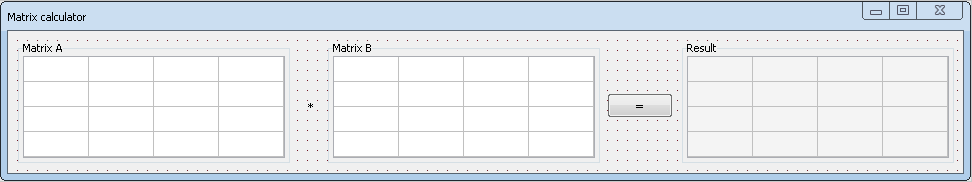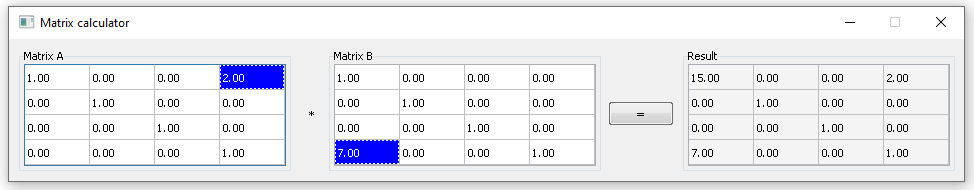## Tutorial: matrix calculator

In this tutorial we will explain what NTL is and how to use it. We also show how to work with StringGrid widget alongside with some other ones you may be familiar from previous tutorials. You will also learn how to compare float numbers properly.

### Introduction

If you look inside Nitisa source code, which you can download right here, you may see there a folder (and a project in the Nitisa solution) called NTL. If you install Nitisa as an extension for Visual Studio it might be difficult to find where the source code is so we recommend to download framework as source code as well. It will help you to observe it and also it has tutorial projects. So, what is NTL? It is Nitisa Template Library. It consists only of template objects and doesn't require compilation. Most of the objects there are mathematical objects like point, rectangle, vector, matrix, plane and so on.

It has a few template constants. Some of them is for π and its variations and some are so called tolerances. You might know that in programming strict comparison does not work for float numbers. The right way to compare them is using some tolerance, like a is equal to b only if they differ not more than by 0.001. Also such a comparison may be absolute or relative. What is the difference? When we talk about absolute comparison we compare numbers using absolute difference between them. In relative comparison we see how many times one value bigger/smaller than another. For example, absolute difference between 1000000 and 1000001 is 1 which is much more than 0.001. So, from the perspective of absolute comparison this values are not equal. In case of relative comparison we see that 1000001 is bigger than 1000000 just about by 1/1000000 = 0.000001 times. So, if we compare them relatively using 0.001 as tolerance we decide they are equal. That is the difference and NTL has two constants defining tolerance used everywhere for absolute and relative comparison. They are Tolerance and RelativeTolerance.

Of cause NTL has helper functions for both relative and absolute comparison. All absolute comparison functions starts from Is. For example, IsLess, IsNotEqual, IsZero, IsBetween and so on. Functions for relative comparison are without Is. They are Equals, NotEquals, Greater and so on.

It should be noted that absolute comparison works much faster than relative one and in most cases it gives expected result but not always. When values are big, like in the example above, or small, the right choice is to use relative comparison.

Besides comparison functions NTL has functions for working with vectors, matrices and other mathematical objects. For example, Inverse functions build inverted matrices, planes, quaternions. Functions which starts by Mat2, Mat3 and Mat4 can be used to build 2D, 3D and 4D matrices on the fly. There are also general utility functions like Abs (return absolute value of the argument), Swap (exchange argument values), Min (return minimum value of arguments) and so on.

Nitisa Template Library also describes mathematical (and others) objects like 4x4 matrix, 4D vector, complex number, plane and so on. Some of them, the most used ones, has aliases in the Nitisa core. For example, Mat4f represents matrices and is defined as `ntl::TMat4<float>`.

That is what NTL is. In this tutorial we are going to create a simple matrix calculator. There are a lot of things that may be done with matrices. In this tutorial we will only implement one operation: multiplication. Of cause matrices in the NTL have much more operations, like calculation determinant, getting minors, finding inverted and transposed matrix, transforming vectors, calculating perspective and orthographic projection matrices and so on. We will also implement our calculator for 4x4 matrices. If you wish, you may later create more flexible one using NxM matrices with TMatrix template.

At first create Nitisa Windows Application project if you use Nitisa for Visual Studio extension or create a new project and a form as described in Tutorial: "Todo list" application (standalone).

### Form layout

Lets now create a form which will look like the one on the image below.To create such a form do the following.

1. Change form's Name property to FormMain.
2. Change form's Caption property to Matrix calculator.
3. Change form's HasMaximizeBox property to false.
4. Change form's HasSizeBox property to false.
5. Change form's WindowPosition property to ScreenCenter.
6. Add widget GroupBox to the form.
7. Change widget's Caption property to Matrix A.
8. Change widget's UseMask property to false.
9. Add widget StringGrid to the group box.
10. Change widget's Name property to StringGridA.
11. Change widget's Align property to Client.
12. Change widget's Columns property to 4.
13. Change widget's FixedColumns property to 0.
14. Change widget's Rows property to 4.
15. Change widget's FixedRows property to 0.
16. Change widget's Multiselect property to false.
17. Add widget Label to the form.
18. Change widget's Caption property to *.
19. Add widget GroupBox to the form.
20. Change widget's Caption property to Matrix B.
21. Change widget's UseMask property to false.
22. Add widget StringGrid to the group box.
23. Change widget's Name property to StringGridB.
24. Change widget's Align property to Client.
25. Change widget's Columns property to 4.
26. Change widget's FixedColumns property to 0.
27. Change widget's Rows property to 4.
28. Change widget's FixedRows property to 0.
29. Change widget's Multiselect property to false.
30. Add widget Button to the form.
31. Change widget's Name property to ButtonCalculate.
32. Change widget's Caption property to =.
33. Generate name for widget's event called OnClick.
34. Add widget GroupBox to the form.
35. Change widget's Caption property to Result.
36. Change widget's UseMask property to false.
37. Add widget StringGrid to the group box.
38. Change widget's Name property to StringGridResult.
39. Change widget's Align property to Client.
40. Change widget's Columns property to 4.
41. Change widget's FixedColumns property to 0.
42. Change widget's Rows property to 4.
43. Change widget's FixedRows property to 0.
44. Change widget's Enabled property to false.
45. Put all widgets in the similar position they are on the picture above using drag and drop and arrow buttons (for more precise position and size change).

### Form logic

Create header file with name FormMain.h in the form folder as usually. Put following code into it.

``````#pragma once

#include "IFormMain.h"

namespace nitisa
{
namespace app
{
class CFormMain :public IFormMain
{
private:
void MatrixToWidget(const Mat4f &m, standard::CStringGrid *widget);
bool WidgetToMatrix(standard::CStringGrid *widget, Mat4f &m);
protected:
void ButtonCalculate_OnClick(IControl *sender) override;
public:
CFormMain();
};

extern CFormMain *FormMain;
}
}``````

In the same folder create source code file FormMain.cpp with the following code.

``````#include "Nitisa/Interfaces/IApplication.h"
#include "Nitisa/Interfaces/IDialogs.h"

#include "Platform/Core/Renderer.h"
#include "Platform/Core/Window.h"

#include "FormMain.h"

namespace nitisa
{
namespace app
{
CFormMain *FormMain{ nullptr };

CFormMain::CFormMain() :
IFormMain(CWindow::Create(), CRenderer::Create())
{
MatrixToWidget(Identity, m_pStringGridA);
MatrixToWidget(Identity, m_pStringGridB);
}

void CFormMain::MatrixToWidget(const Mat4f &m, standard::CStringGrid *widget)
{
for (int row = 0; row < 4; row++)
for (int col = 0; col < 4; col++)
widget->setCell(col, row, ToFixed(m[row][col], 2));
}

bool CFormMain::WidgetToMatrix(standard::CStringGrid *widget, Mat4f &m)
{
for (int row = 0; row < 4; row++)
for (int col = 0; col < 4; col++)
if (!TryStringToFloat(widget->getCell(col, row), m[row][col]))
{
Application->Dialogs->Error(L"You have incorrect number in row " + ToString(row) + L" and column " + ToString(col));
widget->setCellActive(col, row, true);
widget->setCellFocused(col, row, true);
widget->setCellSelected(col, row, true);
return false;
}
return true;
}

void CFormMain::ButtonCalculate_OnClick(IControl *sender)
{
Mat4f a, b;
if (!WidgetToMatrix(m_pStringGridA, a))
return;
if (!WidgetToMatrix(m_pStringGridB, b))
return;
MatrixToWidget(a * b, m_pStringGridResult);
}
}
}``````

Here we added two helper methods. The first one is MatrixToWidget. It takes matrix and StringGrid widget as arguments and put matrix elements into corresponding cells of the widget. The second method is WidgetToMatrix. It takes values from widget cells, convert them into numbers and put into corresponding matrix elements. If cell doesn't contain proper number it shows an error message and method returns false.

There is no way to edit StringGrid cells in the Form Builder so we set default values in form's constructor using helper method MatrixToWidget and Identity constant matrix.

In the method processing button's OnClick event we are getting values from both StringGrid widgets and, if success, calculate matrix product and put into the third StringGrid widget labeled as Result.

Here you can see how working matrix calculator application looks like.You can find project MatrixCalculator with lots of comments in the latest release of the Nitisa framework in `Tutorials\MatrixCalculator` folder of the Nitisa solution. To build it you have to select Debug configuration and x86 platform.

#### Tutorial: simple network chat

If you like Nitisa project consider support it with a small donation or chose one of the paid plan to support further development.